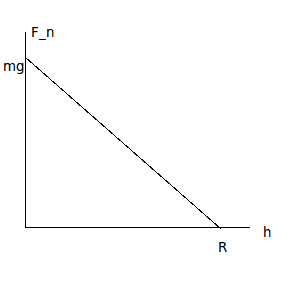# Frictional work inside a circle

StephenP

## Homework Statement

You push an object of mass m slowly, partway up a loop-the-loop track of radius R, starting from the bottom, where the normal force to the track is vertically upward, and ending at a point a height h< R above the bottom. The coefficient of friction between the object and the track is a constant $$\mu$$.
Show that the work you do against friction is $$\mu mg(2rh-h^2)^{1/2}$$

## Homework Equations

$$W=\vec{F}\cdot\vec{x}$$
$$F_f=\mu mg$$

## The Attempt at a Solution

I assume that since the mass is being moved 'slowly', I can ignore the usual equations of circular motion, centripetal force, etc.Further, I noted that at point "A", the normal force is exactly equal/opposite to mg, and that at point "B", the normal force is exactly 0. (at h=r).
I further assumed (not sure if this is valid) that the normal force would decrease linearly from mg to 0 as h goes from 0 to r.Using the basic equation of a line, I get:
$$F_n(h) = \frac{-\mu mg}{r}(h) + \mu mg$$

This is where I more or less run out of steam. I know that I ought to integrate it, but for the life of me I can't figure out how to integrate it "along" the displacement (which would be given by the circle). Simply taking the indefinite integral wrt h yields:
$$\mu mg(\frac{2rh-h^2}{2r})+C$$
Which is rather similar, but obviously significantly different. I guess what I need is a last nudge to figure out what I'm doing wrong, I feel very close but have just gotten frustrated working on this for so long :(
-Stephen

Homework Helper
The normal force doesn't change linearly.
Pick a point on the track, say where your green line meets it, and show mg down. Extend the green line so you can find the component of mg along the green line that is the normal force at that point. Call the angle between the vertical and the green line θ and mark it in two places - the middle of the circle and between mg and the green line.
Once you have an expression for the normal force at angle θ, you can write one for the friction force Ff.

dW = Ff*dL, where dL is a bit of distance along the circular track. Write dL = R*dθ and you should have a dW as a function of θ that you can integrate from 0 to Φ, the angle when the mass is at height h.

I did quite a bit of messing around (including a Pythagorean formula) to get a nice expression for sin(Φ) in terms of R and h to evaluate the integral.

Interesting problem!

StephenP
I had considered more or less the same thing before, but didn't succeed at first and started REALLY overthinking things. Between you and a night's sleep, it all seems rather simple now. Thanks for putting me back on track!Homework Helper
Most welcome! Good luck on the next one.